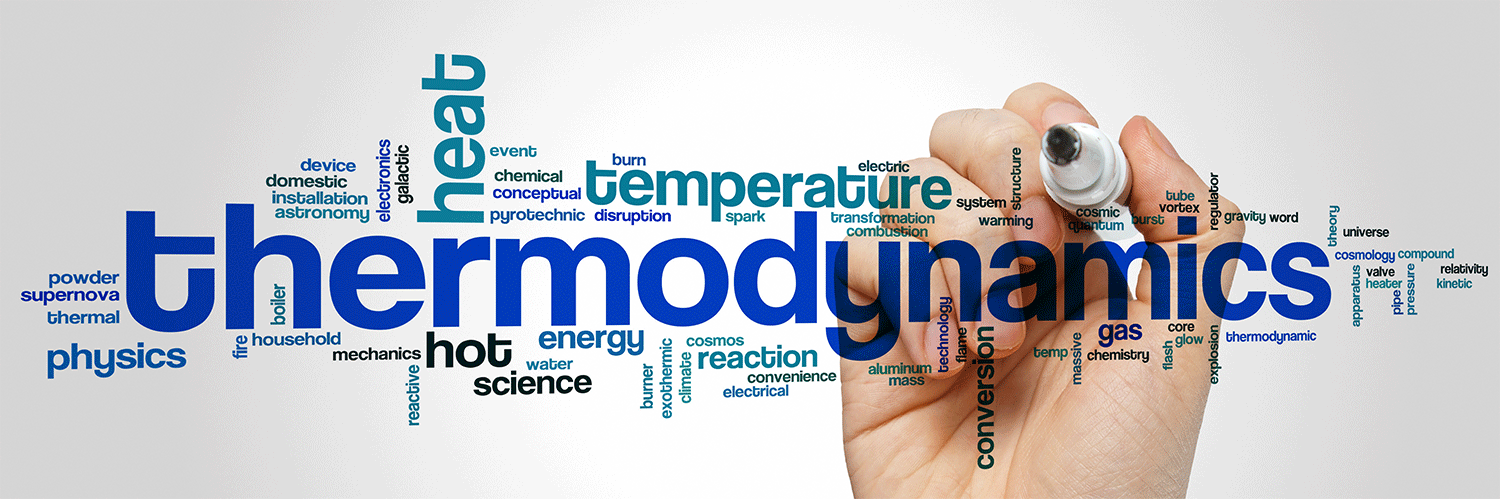# Cryogenic Thermodynamics

For those in the Cryogenic field, thermodynamics is a critical element that affects the delivery of cryogenic liquids and keeps things… well, cryogenic. It is recommended to review the fundamental laws of Thermodynamics that apply to cryogenic liquids first.  There are three basic laws of thermodynamics:

## First Law of Thermodynamics

Energy can be exchanged between the system of interest and its surroundings.  However, the total energy of the system plus the surroundings is constant.  That’s the First Law of Thermodynamics.  The First Law is also stated as energy is conserved.

This is an empirical law, which means that we know that energy is conserved because of many repeated experiments by scientists.  It’s been observed that you can’t get any more energy out of a system than you put into it.  James Prescott Joule did a famous experiment that demonstrated energy conservation and showed that heat and work were both of the exact nature: energy.  His system of interest was water in a thermally insulated container.  In this container was also a paddle connected to the outside world (surroundings) and weights on a string.  Joule measured the work done by the paddle wheel and the heat created by the wheel turning in the water.

Significantly, Joule found that the amount of energy done as work was converted exactly to heat.  The energy was changed from one form to another (work to heat); however, no net change of energy in the system plus the surroundings occurred.  Energy is conserved.

## Second Law of Thermodynamics

The second law concerns entropy (S), a measure of disorder. The second law says that the entropy of the universe increases. If the volume and energy of a system are constant, then every change to the system increases entropy. If volume or energy changes, then the system’s entropy can decrease. However, the entropy of the universe does not decrease. The molecules in one’s body exist in excellent order; this only happens because the entropy of the rest of the universe is increased to a more significant amount than the entropy of the body is decreased. An increase in disorder (overall) is, therefore, spontaneous.

The laws of thermodynamics were determined empirically (by experiment). They are generalizations of repeated scientific experiments. The second law is a generalization of experiments dealing with entropy — it is that the system’s S plus the surroundings’ S is equal to or greater than 0. Entropy is not conserved, like energy.

## Third Law of Thermodynamics

The third law of thermodynamics states that the entropy of a pure, perfect crystal is 0 at 0K: S(0K) = 0.  At 0K, the atoms in a pure, ideal crystal are aligned perfectly and do not move.  Moreover, there is no entropy of mixing since the crystal is pure.  For a mixed crystal containing the atomic or molecular species A and B, there are many possible arrangements of A and B, and there is, therefore, entropy associated with the arrangement of the atoms/molecules.